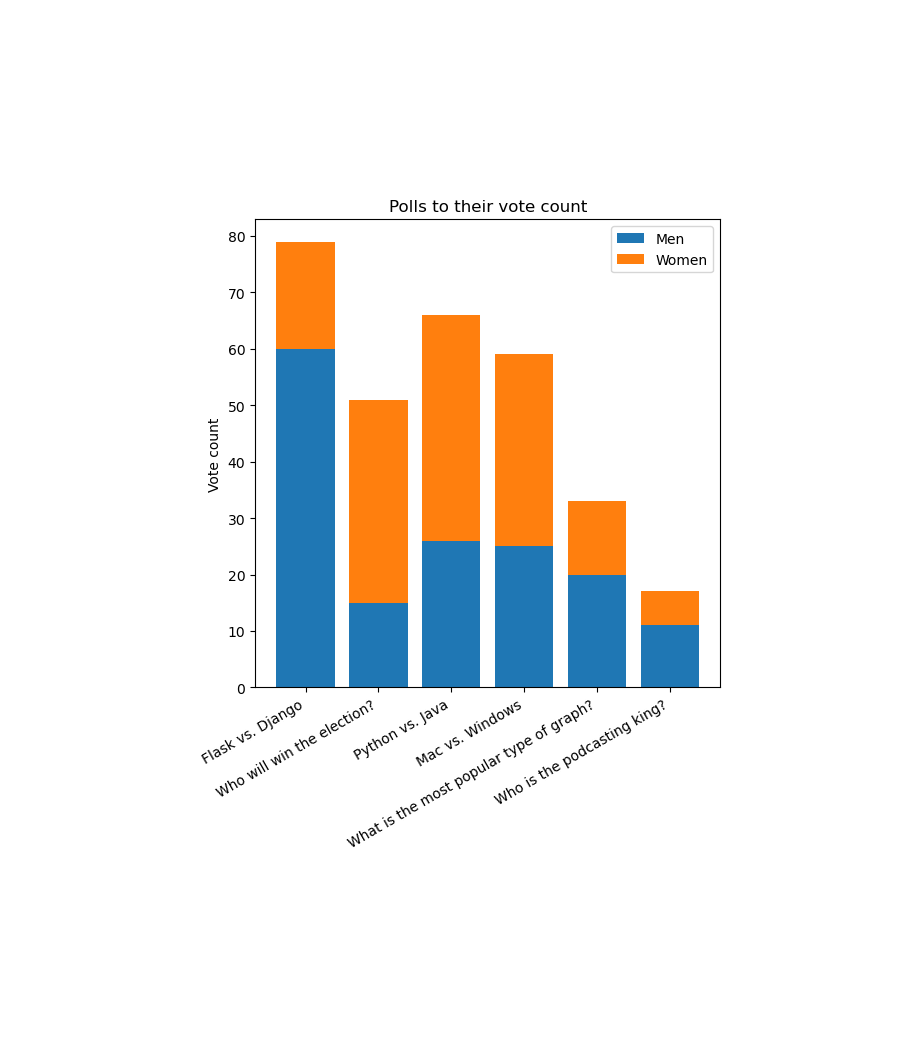# # How to export an image with matplotlib

Instead of `figure.show()` you can use `figure.savefig("graph.png")` (or any other filename!) to create an image file.

There's a couple things you can add as arguments to `.savefig()`:

• `facecolor` and `edgecolor` allow us to set the colour of the main figure or of the edges around the figure. Default to `"white"`.
• `bbox_inches` allows us to set the size of the figure that we want to save. By default it uses the calculated bounding box of the figure. We can set it to a number, in inches, or most commonly to `"tight"` to calculate the size of the figure before exporting the image.
• `pad_inches` allows us to pass in a padding around the figure if `bbox_inches` is set to `"tight"`. By default it's `0.1`.

## #`bbox_inches` and `pad_inches` comparison

Without `bbox_inches` and `pad_inches`, the is exported with its calculated bounding box. This makes use of `subplots_adjust()` and the `figsize` argument:

``````figure = plt.figure((figsize=(6,6))

...

figure.savefig("graph.png")
``````You can alternatively set `bbox_inches="tight"` and `pad_inches` to a number, and then the bounding box will be calculated before exporting.

The exported image may be bigger than the `figsize` to accommodate for axes that would normally be clipped:

``````figure = plt.figure((figsize=(6, 6))

...

``````Note the `pad_inches` is quite large. Skipping `pad_inches` but still setting `bbox_inches="tight"` will still calculate the bounding box, but use the default padding value which is `0.1`:

``````figure = plt.figure((figsize=(6, 6))

...

figure.savefig("graph.png", bbox_inches="tight")
``````## #`facecolor` and `edgecolor` comparison

### #`facecolor`

Produced with `figure.savefig("graph.png", facecolor="#5c44fd")`### #`edgecolor`

Produced with `figure.savefig("graph.png", edgecolor="#5c44fd")`.

The `edgecolor` property is the colour of the border. By default figures have no borders, so this won't be visible unless a border is set with `figure = plt.figure(linewidth=5)`: